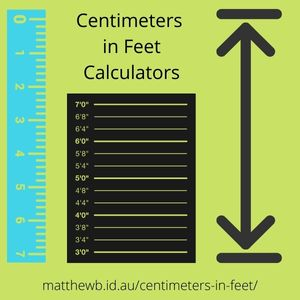## 145 centimeters in feet Calculator

Enter centimeters in the top textbox and click the Get Results button. Results appear below in feet. Remainder is in inches.

Input
centimeters
feet
inches (remainder)

## 145 centimeters in feet Table

centimetersfeetinches (remainder)
10033
10535
11037
11539
120311
12541
13043
13545
14047
14549
150411
15551
16052
16554
17056
17558
180510
18560
19062
19564
20066

## 145 centimeters in feet FAQ

### How do I convert centimeters to feet?

Use the Centimeters in Feet Calculator above. Or use the Centimeters in Feet Table.

### How long is 145 centimeters in feet?

145 centimeters = 4 feet. The remainder is 9 inches. Use the Centimeters in Feet Calculator above.

### How tall is 145cm in feet and inches?

145 cm = 4 feet and 9 inches. Use the Centimeters in Feet Calculator above.

### How tall is 1 meter in feet and inches? 1 meter = 100 centimeters

1 meter = 3 feet and 3 inches. Use the Centimeters in Feet Calculator above.